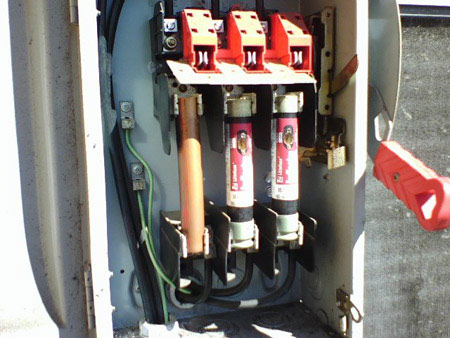# Brainteaser Answers – Issue 13, 2009

In each issue of the Automation Notebook we feature a section of brainteasers. These are the brainteaser answers from 13, 2009 of the Automation Notebook. The brainteaser questions are repeated in black. The answers to the brainteaser questions are highlighted in red with explanations. You can view the brainteasers from Issue 13, 2009 without the answers here: http://library.automationdirect.com/brainteasers-issue-13-2009/

## 1. Think Tank

If the area of the floor of a tank is six square feet, and the tank contains 9 inches of water, how much does the water level rise if a 1 foot cube is placed in the tank? How much farther would it rise if another identical cube is added?

The first block raises the level 1.2 inches for a total of 10.8 inches in the tank. The second block raises it an additional 2.2 inches.

The total amount of water is 6 x 144 x 9 = 7776 cubic inches, this never changes.  When the first block is added, the effective area of the tank changes from 6 square feet to 5 square feet.  So 7776 cubic inches fills the tank to 7776 / (5 x144) = 10.8 inches, a difference of 1.8 inches.  The second block is trickier, because now the water level is higher than 12 inches, and the blocks become submerged.  It takes 4 x 12 x 144 = 6912 cubic inches of water to come even with the top edge of the blocks, leaving 864 cubic inches of water left to spread out across the full 6 square feet (864 square inches).  864 cubic inches of water spread across 864 square inches gives a height of 1 inch. So the final height of the water is 13 inches (12 + 1).  An additional difference of 2.2 inches.

## 2. Close Proximity

An automation vendor has been packaging their proximity sensors in boxes of 160 sensors for years. These small cylindrical parts are typically stacked in 8 rows of 20 sensors each to perfectly fill the boxes. With sales increasing, and production ramping up, the vendor realizes that it may be possible to increase the number of sensors in each box, without changing the box size. How do they do it, and how many more sensors will fit in each box?

Answer: By stacking the sensors in alternating rows of 20 and 19, each box will now accept a 9th row of 20 sensors. The 2nd, 4th, 6th, & 8th rows will only have 19 sensors, so the number of additional sensors is 16 per box (20 – 4).

See this sketch for further clarification:If we define the diameter of a sensor to be one unit, each pair of rows in the new configuration will now stack at √3 or 1.732 units of height.  (the red triangles are identical).  So eight rows will take just over 6.9 units of space, the ninth row completes the stack at 7.93 units (the box was originally defined as holding 8 units).

## 3. What’s Wrong Here? Can You Spot All the NEC Code Violations?